# Sample Paper of Physics

Problem 1.

An athlete participates in a 200 meter race. He or she starts at rest and accelerates at constant acceleration for 5 seconds. After that, the velocity of the athlete is constant until the end. Find the distance and speed of the athlete at the 5th second from the start. Suppose it takes 15 seconds for the athlete to run the entire distance of the race. Find the value of the acceleration.

Solution

The speed of the athlete at the 5th second of the sprint can be found from the equation below:

V=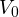+at, whereis initial speed, (=0[m/s]); a is the acceleration, [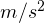]; t is the time of acceleration, (t=5[s]).

Substitution of the above values yields:

V=a*5[]

The distance at the 5th second can be found from the equation below:

r=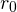+t+1/2a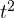whereis the distance at the beginning of the race, (=0 [m]).

Substitution of the above values yields:

r=1/2a*25=12,5a[m]

The entire race can be divided into two sections: the first section with constant acceleration and the second section with constant speed. Since the time of the first section is known and equaled to 5 seconds, the time of the second section is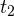=15-5=10 [s].

The distance of the first section, which was found above, is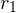=12,5a [m]. The distance of the second section can be found as: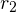=V*=5a*10 [m]. Therefore the whole distance is:

r=+=12,5a+50a=200[m]

From the above equation, the acceleration is:

a=200/62,5=3,2[]

The magnitude of the speed at the 5th second of the race therefore is:

V=5*3,2=16[m/s]

The magnitude of the distance at the 5th second of the race therefore is:

r=12,5*3,2=40[m]

Answer: distance at the 5th second is 40[m], speed at the 5th second is 16[m/s], and acceleration is 3,2 []

Problem 2.

A basketball player tosses the ball from the height of 1.8 meters from the floor. The initial speed of the ball is v0 and the initial angle under which the ball was thrown is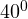vertically, as shown in the picture below. Write a set of equations for the horizontal and vertical positions and velocities of the ball as a function of time. The ball reaches its highest point in 3 seconds. How high is the ball above the ground?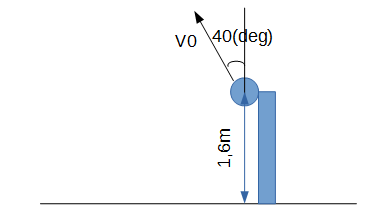Solution

Let us place a two dimensional coordinate system in such a way that the Y axis is a vertical axis and X axis is a horizontal axis, and the origin is the initial location of the ball, as shown in the picture below.

From the picture, the projection of initial speedon the vertical plane is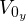=*cos(); the projection of initial speedon the horizontal plane is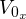=*sin().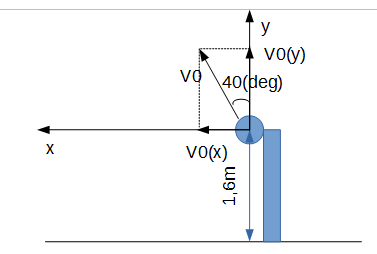The general equation for the position vector depending on time is: r=+*t-1/2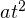, where a is an acceleration of the ball and t is the time.

We can rewrite the above equations as a system of four equations by projecting their components on vertical and horizontal axes: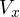==*cos()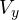=*sin(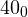)-gt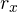=*t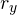=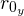+*t-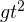/2

At the highest point, vertical velocity is equaled to 0. Therefore, we can find an initial speedas follows:=gt/sin()=10*3/0,643=46,656[m/s]

The distance at the highest point would be:=1,6+*cos()*3-10*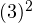/2=1,6+46,656*cos()*3-45=62,82 m

Answer: The initial velocity is 46,656[m/s] and the maximum height above the ground is 62,82[m].

Problem 3.

A ball was thrown down from the bridge at an initial speed of 20 m/s and an angle offrom the vertical axis. At the same time, a boat is passing under the bridge with a speed of 10 m/s, as shown in the picture below. Find the vertical and horizontal components of the initial velocity of the ball as seen by the person on the bridge. Find the vertical and horizontal components of the initial velocity of the ball as seen by the person on the boat. Draw a vector diagram showing how to relate the velocity the ball appears to be moving at as seen from the bridge, as seen by the person in the boat, and the velocity of the boat with respect to the bridge.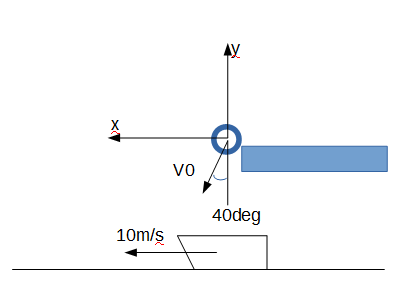Solution

The vertical and horizontal components of the initial velocity of the ball as seen by the person on the bridge are as follows:=*sin()=20*sin()=12,86[m/s]=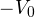*cos()=-20*cos()=-18,79[m/s]

The vertical and horizontal components of the initial velocity of the ball as seen by the person on the boat are as follows: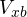=*sin()-10=20*sin()-10=12,86-10=2,86[m/s]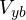=*cos()-0=-20*cos()-0=-18,79[m/s]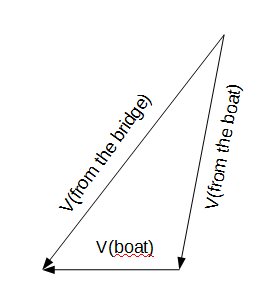We presented you several physics problems with their explanations and posted some of solved physics problems before. Our experts did their best to demonstrate how various formulas and approaches can be used on practice. You can’t use our sample paper of physics as you are probably given another task and your instructor has requirements that differ from those specified above. But you can ask our experts to help you with any type of technical projects and they will be delivered in a timely manner.